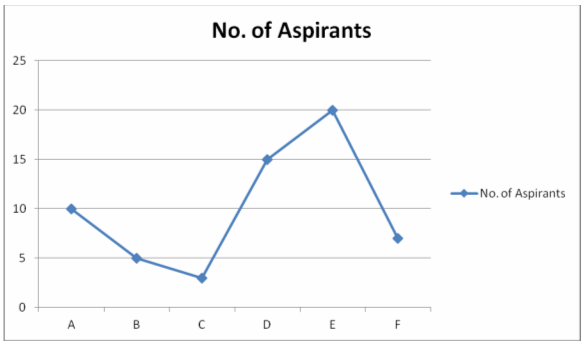# IBPS Quantitative Aptitude model test paper - 2

Home > Aptitude test > Quantitative model test - 2

## IBPS Quantitative Aptitude model test paper - 2

What approximate value should come in place of question mark (?) in the following questions?

Q1. {(45)2 + (652)+(852) } ÷ ? = 50

A. 269
B.2690
C. 0.269
D. 269.5

Q2. 250 + 0.25 /0.5 X 0.005 - ? = 1

A. 250
B. 251
C. 4
D. 249
E. None of these

Q3. {(199.752)- 30000 } ÷ ? = 100000

A. 0.3
B. 4
C. 39 x 107
D. 3900

Q4. 99.09 + 61.9 + 59.19 – 7.19 – 6.91 -7.90 = ?

A. 0
B. 1
C. -1
D. 2

Q5. 12.5% * 33% * 40% * 3333.55 = ?

A. 2350
B. 1250
C. 552
D. 55

Q6. √750.125 x 12.23 % + 6.65 = ?

A. 3.34
B. 12.23
C. 10
D. 17.75

Q7. Rs. 7,50,000 are invested in Rs. 100 shares which are quoted at Rs.1200. Find the income if 25 % dividend is declared on the shares.

A. 1560
B. 15625
C. 15600
D. 16500

Q8. Find the value added tax paid by shopkeeper if he buys a Sofa set for \$ 10000 and pays 7% tax and sells the Sofa set for \$ 13,000 and charges 9% tax.

A. 1170
B. 700
C. 470
D. 1870

Q9. Raju borrowed \$ 2 x 104 for 48 months under some conditions mentioned below :

(i). 10% Simple Interest for the first 30 months.
(ii). 10% Compound Interest for the remaining one and a half years on the amount due after 30 months, the interest being compounded half- yearly.

Find the total amount to be paid at the end of the four years.

A. 25000
B. 28940
C. 65000
D. 31000

Q10. Find the difference between the C.I. and the S.I. on Rs. 4000 @8% p.a. in 2 years.

A. Rs. 15.60
B. Rs. 35.60
C. Rs. 45.60
D. Rs. 25.60

Q11. What will be the perimeter of Semi- Circle of radius 7cm?

A. 77
B. 36
C. 22
D. 13

Directions (Q. 12- 14) Complete the following Series:

Q12. 512, 100, 216, 64 , __ , 36, 8, 16.

A. 64
B. 48
C. 108
D. 96

Q13. 9262, 442, 10650, 490, 12170,___

A. 504
B. 14240
C. 538
D. 532

Q14. 1331, 1000, 729, ___, 343, 216.

A. 1331
B. 675
C. 512
D. 425

Q15. What will be the Rate of Interest? If Salman has a recurring deposit account in a bank. He deposits Rs. 2500/month for 2 years. If he gets Rs. 66250 at the time of maturity.

A. 8.75 %
B. 10%
C. 12.50%
D. 11.50 %

Directions (Q. 16 -20) Study the following graph carefully to answer the questions :

Number of Aspirants Appearing for Banking Exams from various InstitutesQ16. What is the ratio of the number of aspirants appearing for the banking exam from Institute A,C, and E together to the number of aspirants appearing for the exam from Institute B, D and F together?

A. 27/33
B. 9/12
C. 11/9
D. 33/25

Q17. What is the ratio of the number of aspirants appearing for the Banking exam from the Institute C to Institute F?

A. 2/7
B. 3/7
C. 1/2
D. 4/7

Q18. What is the average number of aspirant appearing for Banking exams from all the institutes together?

A. 555555.5
B. 100
C. 833333.33
D. 1000000

Q19. The number of aspirants appearing for exam from Institute F is what percent of the total number of aspirants appearing for exam from all the institutes together?

A. 11.66
B. 13.33
C. 15
D. 6

Q20. The number of aspirants appeared for Banking exam from Institute B is approximately what percent of the number of aspirants appearing for exam from Institute E ?

A. 5
B. 25
C. 33.33
D. 75

Directions (Q.21 -25): Study the following given information carefully and answer the questions that follows:

In a bag there are 2 Yellow, 3 Violet, 6 Black and 4 Blue balls.

Q21. If two balls are picked at random, what is the probability that either both are yellow or both are violet ?

A. 1/9
B. 13/35
C. 7/91
D. 69/91
E. None of these

Q22. If four balls are picked at random, what is the probability that one is yellow, two are blue and one is black ?

A. 24/455
B. 18/455
C. 11/15
D. 9/11

Q23. If 3 balls picked at random, what is the probability that one is violet and two are blue balls?

A. 24/455
B. 18/455
C. 4/105
D. 1/9

Q24. If two balls picked at random, what is the probability that both are black?

A. 2/14
B. 1/9
C. 7/8
D. 1/3

Q25. If four balls are picked at random, what is the probability that at least one is blue?

A. 69/91
B. 14/15
C. 8/9
D. None of these